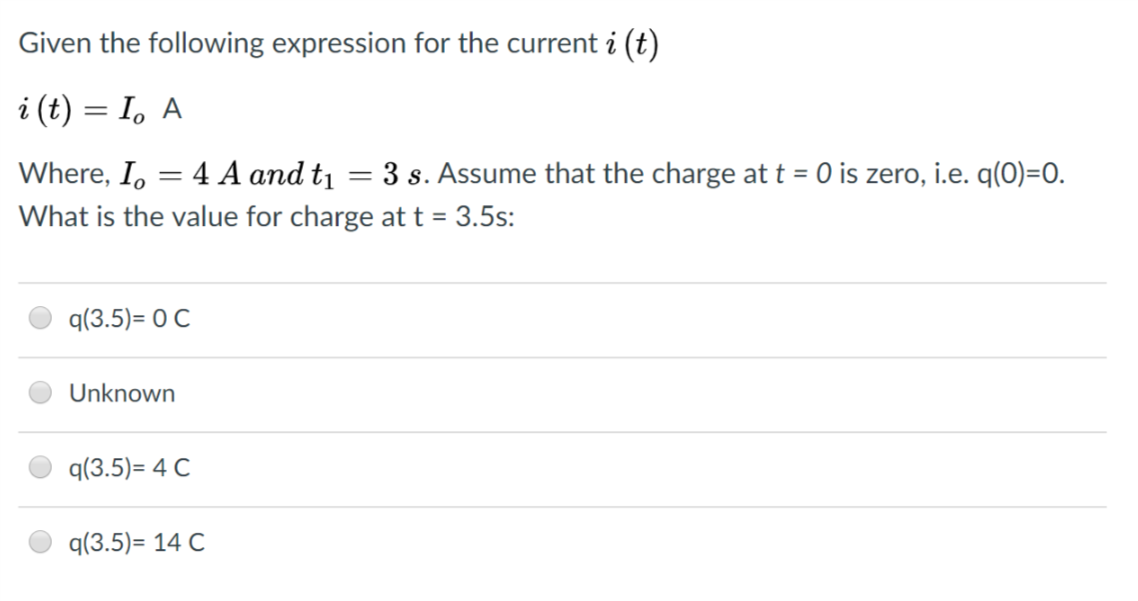# Given the following expression for the current i (t)Where, Io 4 A and tı 3 s. Assume that the charge at t 0 is zero, i.e. q(0)-0.What is the value for charge at t 3.5s:q(3.5)-O CUnknownq(3.5)-4 Cq(3.5)- 14 C

Questionhelp_outlineImage TranscriptioncloseGiven the following expression for the current i (t) Where, Io 4 A and tı 3 s. Assume that the charge at t 0 is zero, i.e. q(0)-0. What is the value for charge at t 3.5s: q(3.5)-O C Unknown q(3.5)-4 C q(3.5)- 14 C fullscreen
check_circleExpert Solution
Step 1

Step-1:

To determine: The value of charge at time t = 3.5 s.

Given information:  The expression of current is

• Current I0=4 A at time t1=3 s.
• At time t=0 charge q(0) is zero.

Step 2

Formula used:

The charge transferred between time t0 to t is obtained by expression:

Here q is the charge, i is the current at time t.

Step 3

Calculation:

Find the charge at time t = 3.5 s:

Substitute the the values i = I0 A, t0 = 0 s and t = 3.5 s in equation-1  to find the charge,

Integrate the function and substitute value of the time...

### Want to see the full answer?

See Solution

#### Want to see this answer and more?

Solutions are written by subject experts who are available 24/7. Questions are typically answered within 1 hour*

See Solution
*Response times may vary by subject and question
Tagged in

### Physics